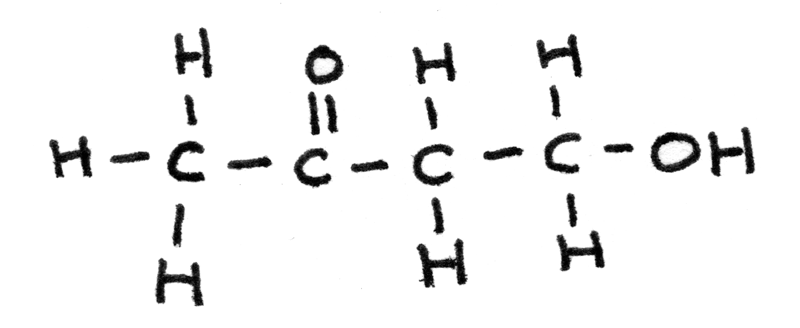# NMR Spectroscopy

Nuclear magnetic resonance (NMR) spectroscopy is an analytical technique used for determining molecular structures. In organic chemistry, proton ($^{1}H^{+}$) NMR and carbon-13 ($^{13}C$) NMR are commonly used. NMR works on the principle of nuclei absorbing and reemitting electromagnetic radiation. A spectrum is produced with peaks corresponding to the atoms in a structure.

### Chemical Shift

Chemical shift $\delta$, is the position on an NMR spectrum at which a particular nucleus absorbs energy. The $\delta$ scale is measured in parts per million ($ppm$).

#### TMS

Chemical shift is measured relative to a single sharp reference signal from tetramethylsilane (TMS) defined to be at $\delta = 0~ppm$. As it is chemically unreactive and volatile, it can be removed from a sample after use.

#### Solvents used for NMR

In NMR spectroscopy, the sample is usually mixed into a solvent. As organic solvents contain hydrogen and carbon, an alternative solvent must be used to reduce unwanted signals on the spectrum.

Deuterium ($D$) is an isotope of hydrogen with one proton and one neutron which due to an even number of neutrons, doesn’t produce a visible signal. $CDCl_{3}$ is therefore commonly used as a solvent. In carbon-13 NMR, the carbon peak can be automatically removed from a spectrum. After the analysis, the solvent can be evaporated to recover the sample.

## Carbon-13 NMR

Carbon-13 NMR is a method used for the identification of carbon atoms in an organic structure. On a spectrum the chemical shift, $\delta$ corresponds to the environment a carbon atom is in. An environment refers to the position and bonded atoms to a carbon atom in a compound. The diagram below shows typical shifts for different environments: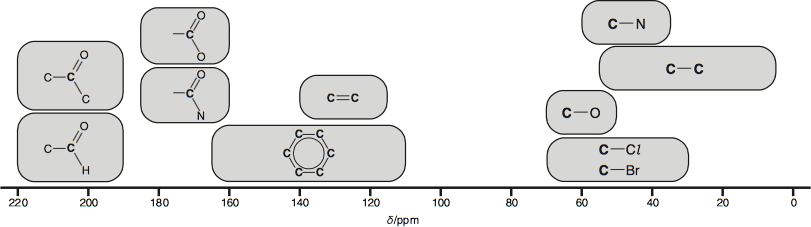The actual values may vary depending on the solvent, concentration and any substituents used.

### Interpreting NMR Spectra

A carbon-13 NMR spectrum shows:

• The number of carbon environments in a structure indicated by the number of peaks.
• The type of carbon environment responsible for each peak. This information can confirm the existence of some functional groups in a compound. On a spectrum this is shown by the chemical shift, $\delta$.

The size or area under a peak doesn’t indicate anything about the number of carbon atoms in a particular environment.

## Carbon Environments

The displayed formula of pronan-1-ol and propan-2-ol are shown below, with the carbon atoms labelled according to the carbon environment they are in.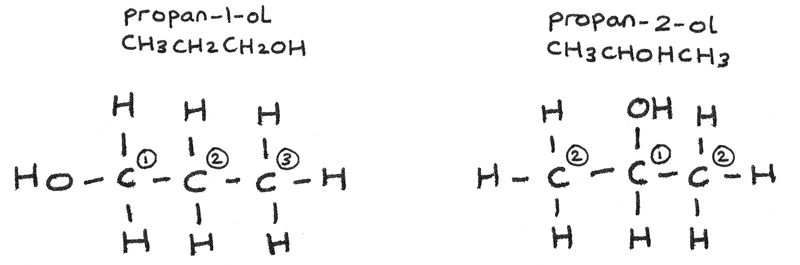• Propan-1-ol has 3 carbon environments so the NMR spectrum will have three peaks.
• Propan-2-ol has 2 carbon environments so the NMR spectrum will have two peaks.

### Type of Carbon Environments

The NMR spectra for propan-1-ol and propan-2-ol are shown below. The carbon environments in the structure are represented by the peaks.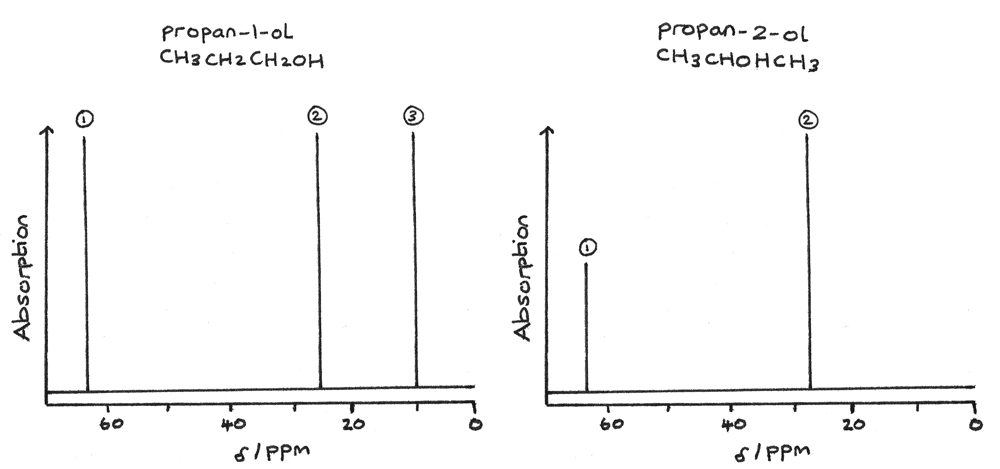Using the known chemical shift values, the type of carbon environment for each peak can be determined. For propan-1-ol, it has three peaks and therefore three carbon environments:

• 1 peak at $\delta = 64~ppm$ for $C-O$.
• 1 peak at $\delta = 15~ppm$ for $C-C$.
• 1 peak at $\delta = 27~ppm$ for $C-C$.

Typically the carbon atom furthest away from the oxygen has the lowest chemical shift. In this example the carbon, $CH_{3}$ at the end of the chain has the lowest chemical shift.

For propan-2-ol, the spectrum has two peaks and therefore two carbon environments:

• 1 peak at $\delta = 64~ppm$ for $C-O$.
• 1 peak at $\delta = 28~ppm$ for $C-C$.

As two carbon atoms are equivalent they are shown as a single peak at $\delta = 28~ppm$ for $C-C$ on the spectrum.

### Proton NMR

A proton NMR spectrum gives the following information about the hydrogen atoms in a structure:

• The number of peaks indicate the number of hydrogen environments in a structure.
• The chemical shift, $\delta$ of the peaks indicate the type of proton environment.
• The relative peak areas indicate the number of hydrogen atoms in a particular environment. This information can be displayed as an integration trace on a spectrum.

The chemical shifts indicate the types of proton environment and functional groups in a compound. A proton NMR spectrum has a narrower range of values for the chemical shift so overlapping of signals may occur.The chemical shift for $O-H$ and $N-H$ can especially vary depending on the concentration and solvent used.

#### Proton NMR Spectrum

The image below shows a NMR spectrum of a compound with the molecular formula $C_{3}H_{6}O_{2}$.• Two peaks on the spectrum indicate that there are two $H$ environments in the compound. As the compound contains 6 hydrogen atoms, some of these must be in the same environment.
• The integration data shows the ratio of hydrogen atoms in each environment is $1:1$, therefore each environment must contain 3 hydrogen atoms.

The chemical shifts indicate the type of proton environment:

• 1 peak at $\delta = 3.7~ppm$ for $O-CH$. As there are 3 hydrogen atoms in this environment the compound must contain $O-CH_{3}$.
• 1 peak at $\delta = 2.2~ppm$ for $OC-CH$. As there are 3 hydrogen atoms in this environment the compound must contain $O=C-CH_{3}$.

By collating this information together the analysed sample must have the structural formula $CH_{3}COOCH_{3}$. The displayed formula is shown below with the hydrogen atoms labelled according to their environment.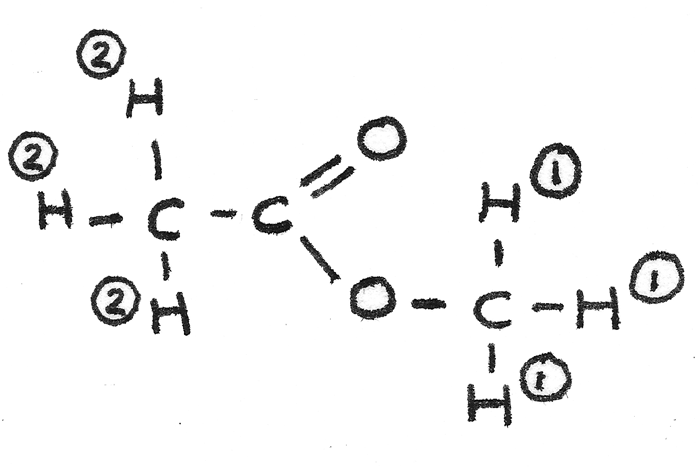### Spin-spin Coupling

Signals in an NMR spectrum may be split into distinctive patterns due to interactions between protons on adjacent carbon atoms. This splitting can indicate the number of proton atoms on adjacent carbons, which assists in deriving a structural formula.

In NMR spectroscopy the number of protons on an adjacent carbon, $n$ corresponds to a peak splitting to form $n + 1$ peaks.

Protons
Splitting Pattern Number of Peaks
0 Singlet 1
1 Doublet 2
2 Triplet 3
3 Quartet 4

The proton NMR spectrum below is of methyl propanoate, $CH_{3}CH_{2}COOCH_{3}$.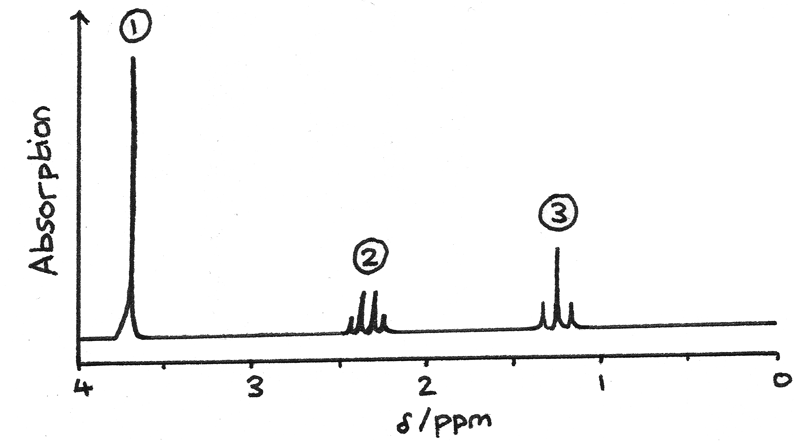• Signal 1 is due to the protons on $O-CH{3}$ . This is a singlet as there are no adjacent protons.
• Signal 2 is due to the protons on $-CH_{2}-$. This is a quartet as there are 3 adjacent protons on $CH_{3}-$.
• Signal 3 is due to the protons on $CH_{3}-$. This is a triplet as there are 2 adjacent protons on $-CH_{2}-$.

Spin-spin coupling occurs between non-equivalent protons so protons on the same carbon atom do not produce their own splitting pattern.

### NMR with -OH and -NH Protons

The protons attached to $-OH$ and $-NH_{2}$ groups are called labile protons. These can be difficult to identify as:

• The peaks can appear over a wide range of possible chemical shift values so they can often be misinterpreted as a different functional group.
• The peaks are often broad and can overlap with other signals. This is due to traces of water in the solvent forming hydrogen bonds with the $-OH$ and $-NH$.
• Usually they produce no splitting pattern for protons on adjacent carbon atoms.

Deuterium oxide ($D_{2}O$) can be mixed with a compound in order to exchange $-D$ in place of the hydrogen atoms on $-OH$ and $-NH$. As $D$ has an even number of nucleons, it produces no visible peak on a spectrum. By comparing the spectrum with $D_{2}O$ to the spectrum without $D_{2}O$, it is possible to identify the peaks generated by $-OH$ or $-NH$ groups.

The NMR spectrum below is for a compound with the molecular formula $C_{3}H_{7}NO_{2}$.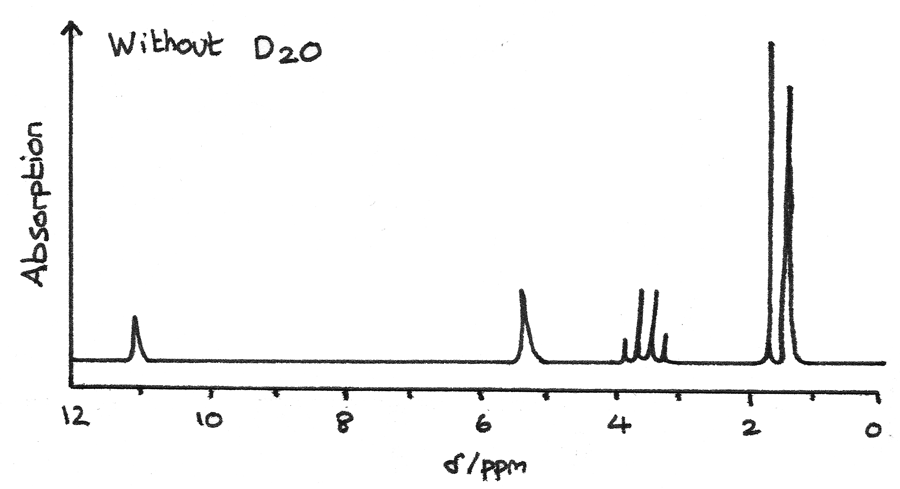The spectrum below is after $D_{2}O$ has been added to the mixture. The missing peaks indicate that they were originally due to $-OH$ or $-NH$ groups.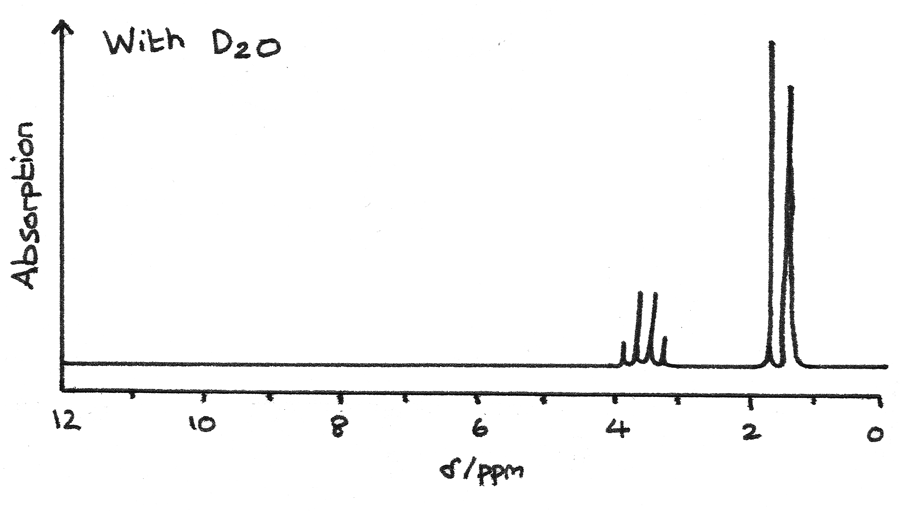### NMR in Medicine

NMR can be used in a medical scanning technique known as magnetic resonance imaging. MRI scanning is a non-invasive and non-destructive method of imaging internal organs.

MRI produces a three dimensional NMR spectrum based on the concentration of hydrogen-containing molecules throughout the body. This information can be used to detect and monitor tumours in organs or to reveal injuries in muscles or tendons.

## Combined Techniques

The three spectroscopy techniques can be combined in order to derive the structure of a sample compound.

#### Mass Spectrometry

• The highest m/z peak indicates the $Mr$ of the compound. If the empirical formula has been determined from chemical analysis, this can reveal the molecular formula of the sample.
• Fragment ions can give clues about sections of the structure.

#### Infrared Spectroscopy

IR gives information about the different types of bonds present in a compound. As different compounds may share the same bonds, IR is often used alongside other analysis techniques.

#### NMR Spectroscopy

Carbon-13 NMR gives information about the number and types of carbon environments in a molecule. Proton NMR gives information about the number and types of hydrogen environments in a molecule. It also provides information about adjacent protons.

Feature Carbon-13 NMR Proton NMR
Number of Peaks Number of $C$ environments Number of $H$ environments
Chemical Shift Type of $C$ environment Type of $H$ environment
Relative Peak Area - Relative number of protons
in each environment
Splitting - Number of protons on adjacent carbon

## Worked Example

The empirical formula of an unknown compound is found to be $C_{2}H_{4}O$. Use the information below to determine the structure of the compound.

1. First the IR spectrum can reveal functional groups in a compound. The very broad peak at $3200-3550~cm^{-1}$ indicates a $O-H$ bond found in alcohols while the sharp peak at $1640-1750~cm^{-1}$ indicates a $C=O$ bond found in aldehydes or ketones.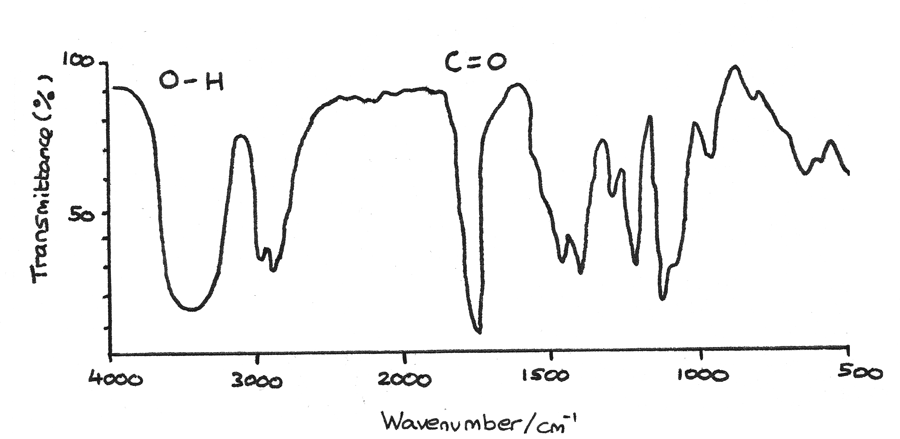2. Next mass spectroscopy can determine the molecular mass and therefore the molecular formula of a compound. The highest peak on the spectrum is $m/z = 88$, therefore the $Mr = 88$. As the empirical formula has mass $44$, the molecular formula must be $C_{4}H_{8}O_{2}$. The peak at $m/z = 44$ could be due to a $CH_{3}O$ fragment ion.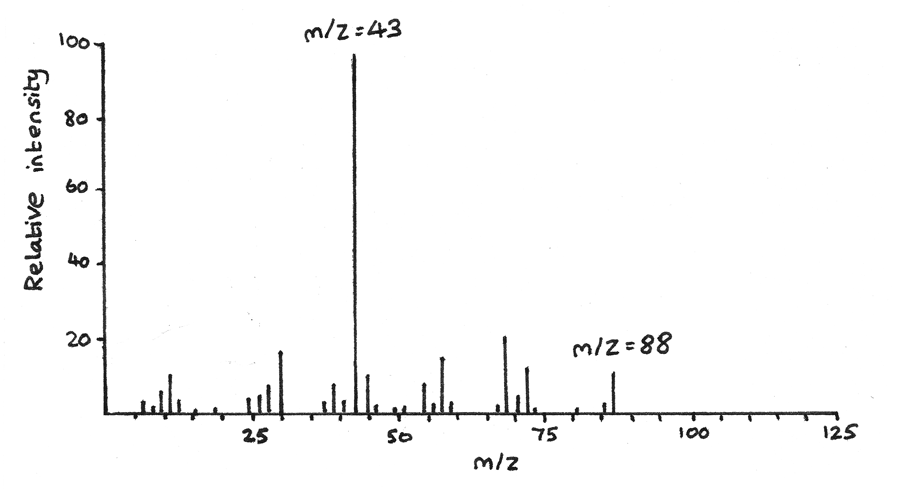3. The proton NMR gives information about the hydrogen atoms and their environments in the structure.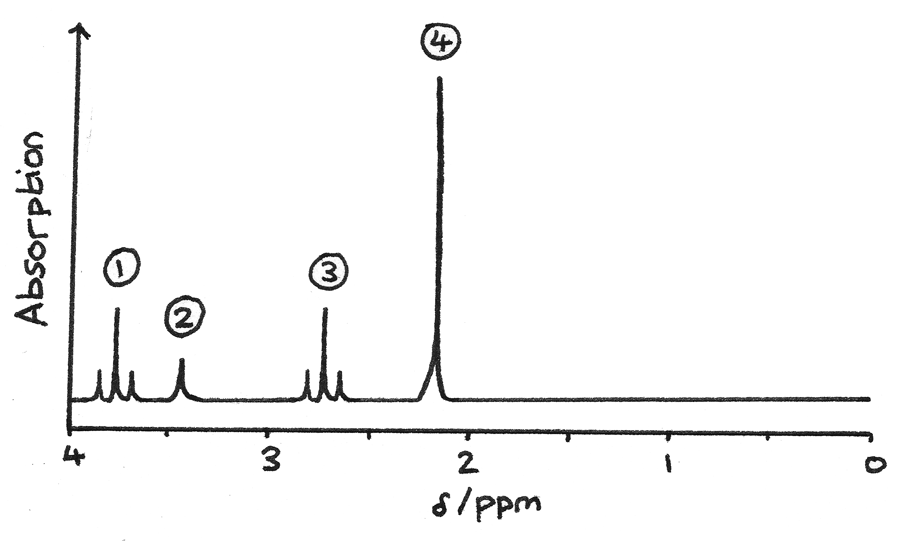• Signal $1$ is a triplet which could be due to $O-CH$, with two adjacent protons.
• Signal $2$ is a singlet which could be due to $O-H$, with no adjacent protons. This could be confirmed by using $D_{2}O$.
• Signal $3$ is a triplet most likely due to $R-CH$ hydrogen atoms, with two adjacent protons.
• Signal $4$ is a singlet due to $HC-CO$, with no adjacent protons.

By combining this information, the actual structure can be determined: